# AutoCLIP Auto-tuning Zero-Shot Classifiers for Vision-Language Models

This is my reading note for AutoCLIP: Auto-tuning Zero-Shot Classifiers for Vision-Language Models. This paper proposes a method to use clip for zero shot image classification, to do that, it first generates several prompt to convert class label to text embedding by average. Then the image is processed by visual encoder. The label of image is the one has slowest distance between label embody and image embedding. This paper propose to use soft Max instead of average for label embedding.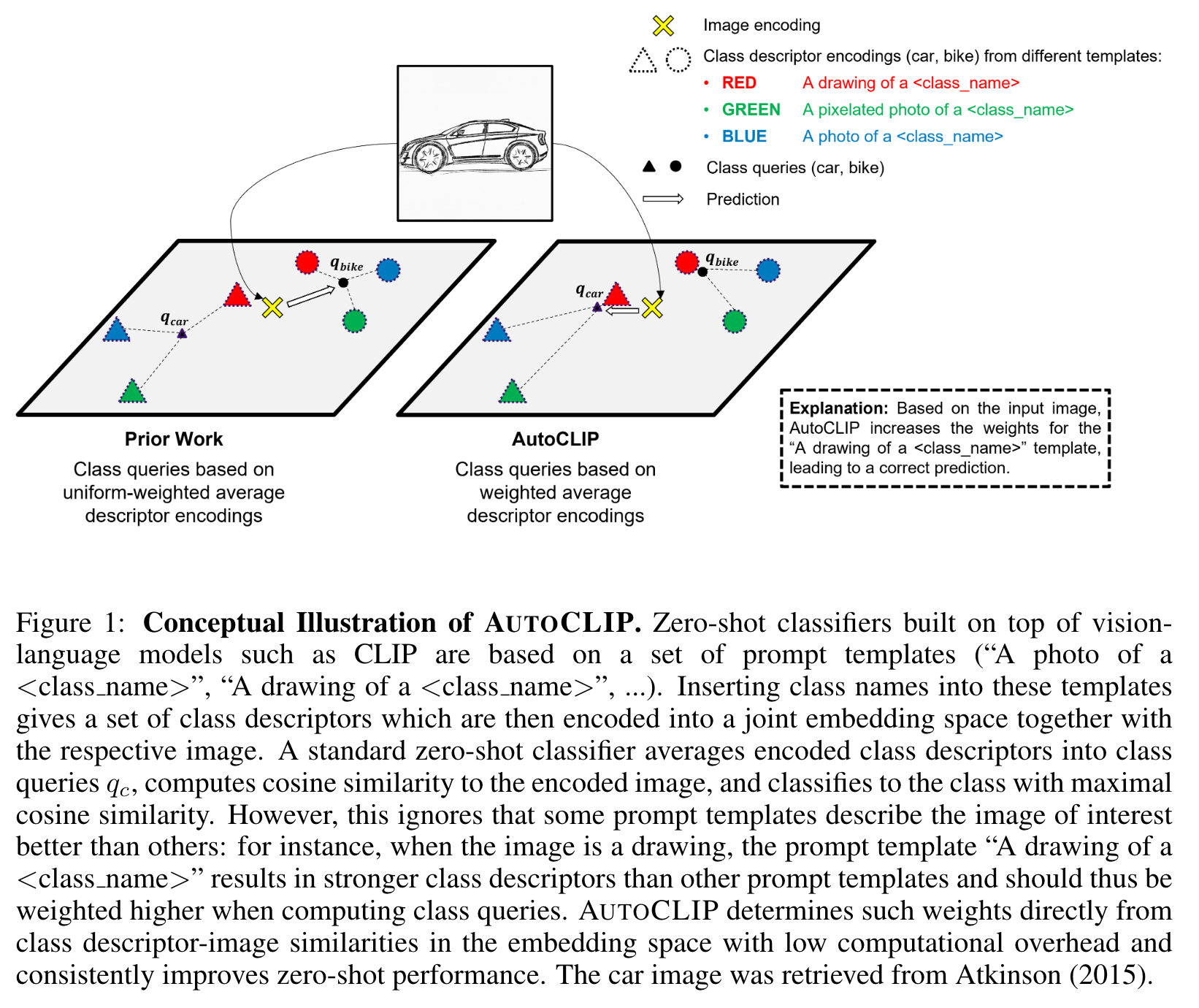# Introduction

In contrast, deriving zero-shot classifiers from the respective encoded class descriptors has remained nearly unchanged, that is: classify to the class that maximizes the cosine similarity between its averaged encoded class descriptors and the encoded image. However, weighting all class descriptors equally can be suboptimal when certain descriptors match visual clues on a given image better than others. In this work, we propose AUTOCLIP, a method for auto-tuning zero-shot classifiers. AUTOCLIP assigns to each prompt template per-image weights, which are derived from statistics of class descriptorimage similarities at inference time. AUTOCLIP is fully unsupervised, has very low overhead, and can be easily implemented in few lines of code. (p. 1)

However, the proposed TPT methods come with substantially increased inference cost because for every input, several image augmentations are required that need to be processed by the image encoder. Moreover, gradients with respect to prompts require backpropagating through the text encoder. (p. 1)

In contrast, we propose tuning not the prompts at inference/test-time, but rather using a large set of predefined and fixed prompt templates whose weighting is adapted. This has the advantage that adaptation can happen in embedding space, without requiring additional forward or backward passes through the encoders, which greatly decreases computation and memory overhead. (p. 1)

We briefly summarize the standard way of constructing zero-shot classifiers on top of VLMs (see Figure 1 left): by instantiating the prompt templates for the respective class, a set of class descriptors is obtained. These descriptors are processed by the text encoder and the resulting encoded descriptors are averaged to obtain image-independent class queries. The image to be classified is encoded by the image encoder and the cosine similarity of the encoded image to every (averaged) class query is computed. The image is then assigned to the class with maximal similarity. (p. 1)

Our proposed method AUTOCLIP changes this way of constructing zero-shot classifiers. AUTOCLIP does not simply uniformly average the encoded class descriptors, but takes a weighted average, wherein weights are automatically tuned for every image separately. Weights are determined such that prompt templates whose resulting class descriptors are closer to the embedding of the respective image get higher weights than those being less similar (see Figure 1 right). This is motivated by the intuition that class descriptors whose encodings are more similar to the encoded image correspond to text prompts that describe the image better than ones with lower similarity (p. 2)

# Related Work

## Vision-Language Pretraining

CLIP is one of the state-of-the art VLMs pretrained on the well-curated WebImageText dataset containing 400 millions image-text pairs using a contrastive loss (Radford et al., 2021). In terms of datasets used, ALIGN requires less dataset preprocessing enabling training on a dataset of over a billion image-text pairs (Jia et al., 2021). Florence (Yuan et al., 2021) expands models to other common modalities (e.g., videos). In terms of the training loss, CoCa (Yu et al., 2022) leverages an additional captioning loss allowing models to be used in generative applications. In our work, we study how to optimally use text prompts of the target classes with these VLMs (p. 3)

## Prompt Construction

Conventionally, one or several manually designed text prompts per target class are employed for zero-shot classification (Radford et al., 2021; Jia et al., 2021). Recent research demonstrates that introducing additional prompts can improve overall performance. DCLIP (Menon & Vondrick, 2022) generates additional prompts based on querying the large-language model GPT-3 (Brown et al., 2020). WaffleCLIP has shown that classification performance can be further boosted by appending random words or characters to predefined prompt templates (Roth et al., 2023). To derive a zero-shot classifier, these works weight all text prompts equally (uniform weighting). (p. 3)

TENT (Wang et al., 2020) demonstrates that adapting models to minimize prediction entropy can improve model performance at test time. In the context of VLMs, TPT (Shu et al., 2022) optimizes prompts of target classes based on the entropy minimization objective. RLCF (Zhao et al., 2023) demonstrates that minimizing the entropy objective can lead to overfitting under distribution shift and proposes adaptation based on average CLIP scores. (p. 3)

# AUTOCLIP

## BACKGROUND

The default zero-shot classifier for a VLM is summarized in Algorithm 1: average class descriptor encodings e(d) into class queries qj , compute cosine similarities sj between class query and encoded image e(x), and classify to the class that maximizes cosine similarity. (p. 3)

## AUTO-TUNING ZERO-SHOT CLASSFIERS

AUTOCLIP uses a weighted average: $q_j = \sum^K_{i=1} {w_i e^{(d)}{ij}$ with learnable w satisfying $w_i \geq 0, \sum^K{i=1}{w_i = 1}$ (p. 3)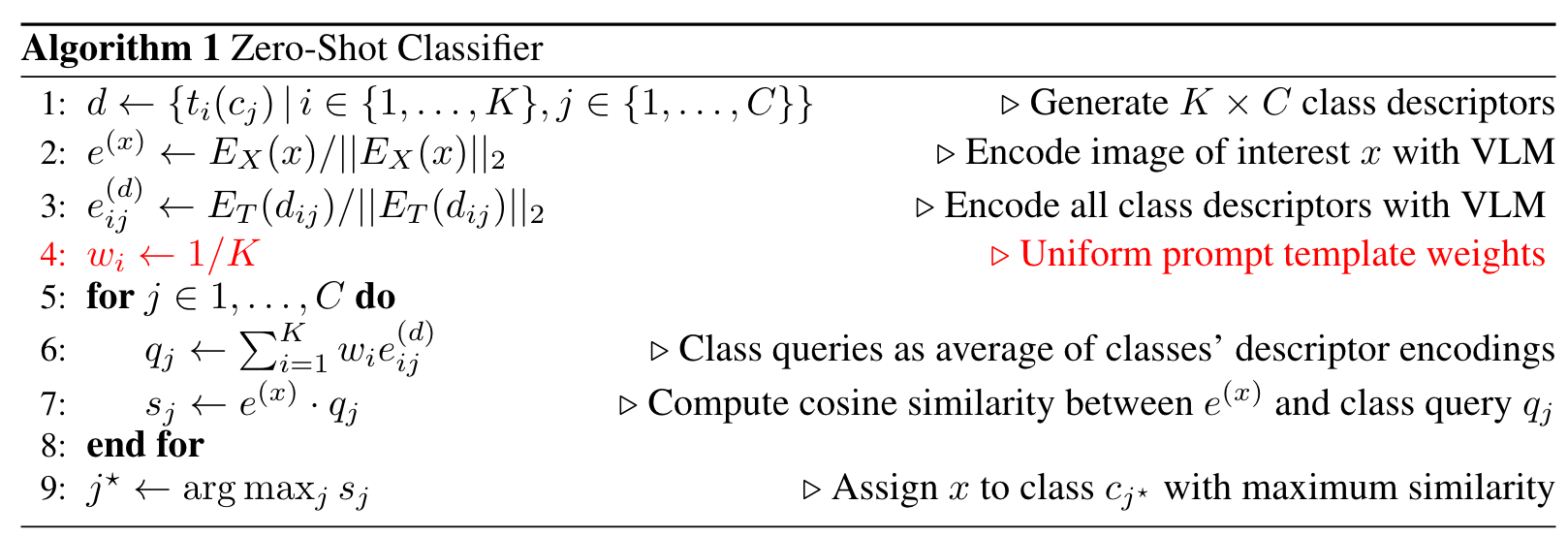AUTOCLIP’s guiding intuition (see Figure 1) is to assign higher weights wi to prompt templates ti that result in class descriptor encodings $e^{(d)}_{ij}$ that are more similar to the encoded image e^(x), since similarity in embedding space corresponds to a class descriptor better describing the image (according to contrastive pretraining objectives in typical VLMs) (p. 4)

For each prompt template ti, we get C class descriptor encodings $e^{(d)}{ij}$ (j = 1, . . . , C) whose similarities $e^{(xd)}{ij}=e^{(d)}{ij}\times e^(x)$ to the the encoded e(x) need to be aggregated when determining the templates’ weighting. Intuitively, simply averaging all classes’ similarities (“mean” aggregation) ignores that in classification we ultimately only care about classes that result in the descriptors closest to e(x); however, taking only the class with highest similarity per template into account (“max” aggregation) ignores inherent ambiguity in the image and was found to be suboptimal (Roth et al., 2023). We propose a middle ground of aggregating via a smooth approximation to the maximum function via $\mbox{logsumexp}e^{(xd)}{ij}=\log{\sum{e^{e^{(d)}{ij}}}}$. This logsumexp aggregation takes all classes into account but assigns higher importance to more relevant classes (ones resulting in higher similarities to the image x). AUTOCLIP then determines weights wi such that $\mbox{logsumexp}w_i e^{(xd)}{ij}=\mbox{logsumexp}(w\times e^{(xd)}=\mbox{logsumexp}(\mbox{softmax}(\rho) e^{(xd)}$ gets increased by one step of gradient ascent in the direction of $\Delta_\rhi\mbox{logsumexp}(\mbox{softmax}(\rho) e^{(xd)}$. We note that − logsumexp has been interpreted as the energy function of a data point (for appropriately trained classifiers) (Grathwohl et al., 2020); in this view, AUTOCLIP can be interpreted as minimizing the energy and maximizing the probability density p(x) of x under the zero-shot classifier. (p. 4)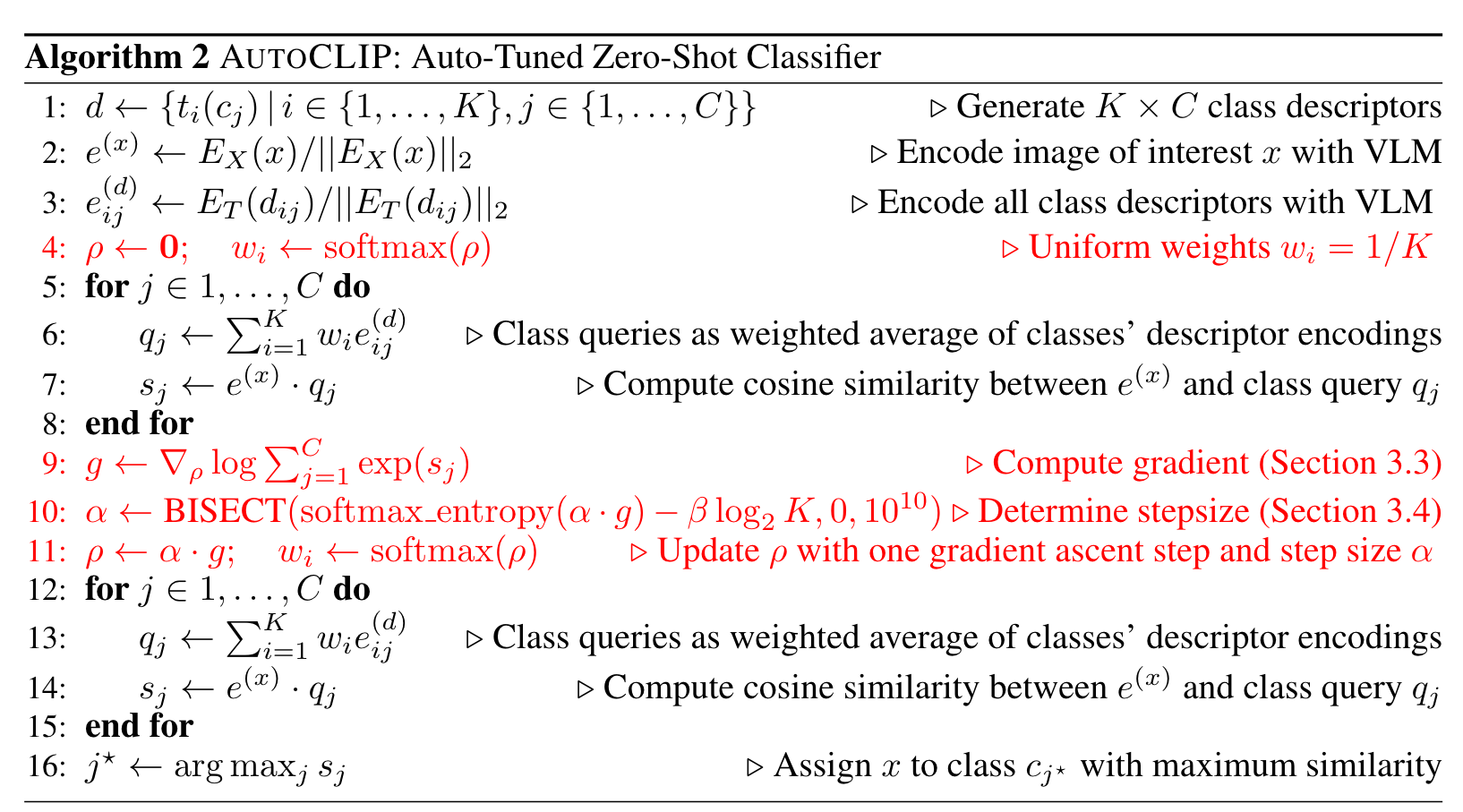For such cases, the gradient ∇ρ logsumexp(s) can also be computed in closed-form: $\Delta_\rhi\mbox{logsumexp}(\mbox{softmax}(\rho) e^{(xd)}=\sum_^K_{k=1}(\sum_{j=1}^C\mbox{softmax}(s)j \times e^{xd}{ij})w_i(\delta_{i,j}-w_k)$, with δ_ij being the Kronecker delta function with δ_ii = 1 and δ_ij = 0 for i ̸= j (p. 5)

## AUTO-TUNING THE STEP SIZE

that we set globally to β = 0.85. Intuitively, β → 1 corresponds to more equally weighted prompt templates while β → 0 to selecting the prompt template with maximum similarity (p. 5)

# EXPERIMENTS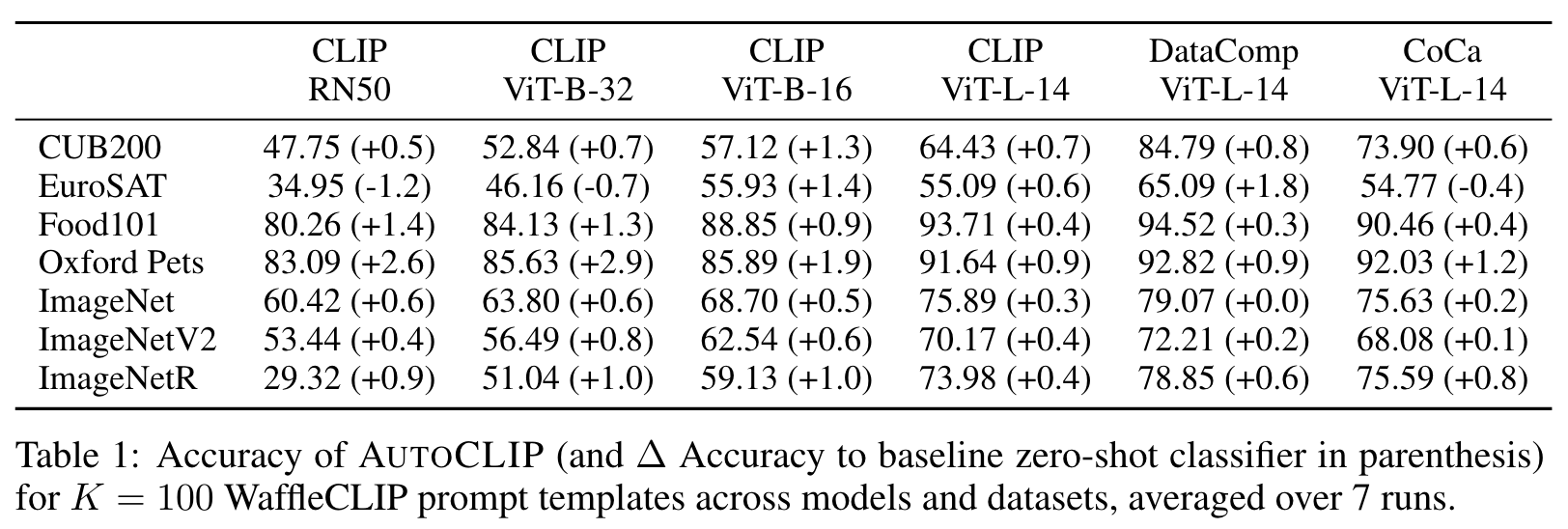# Ablations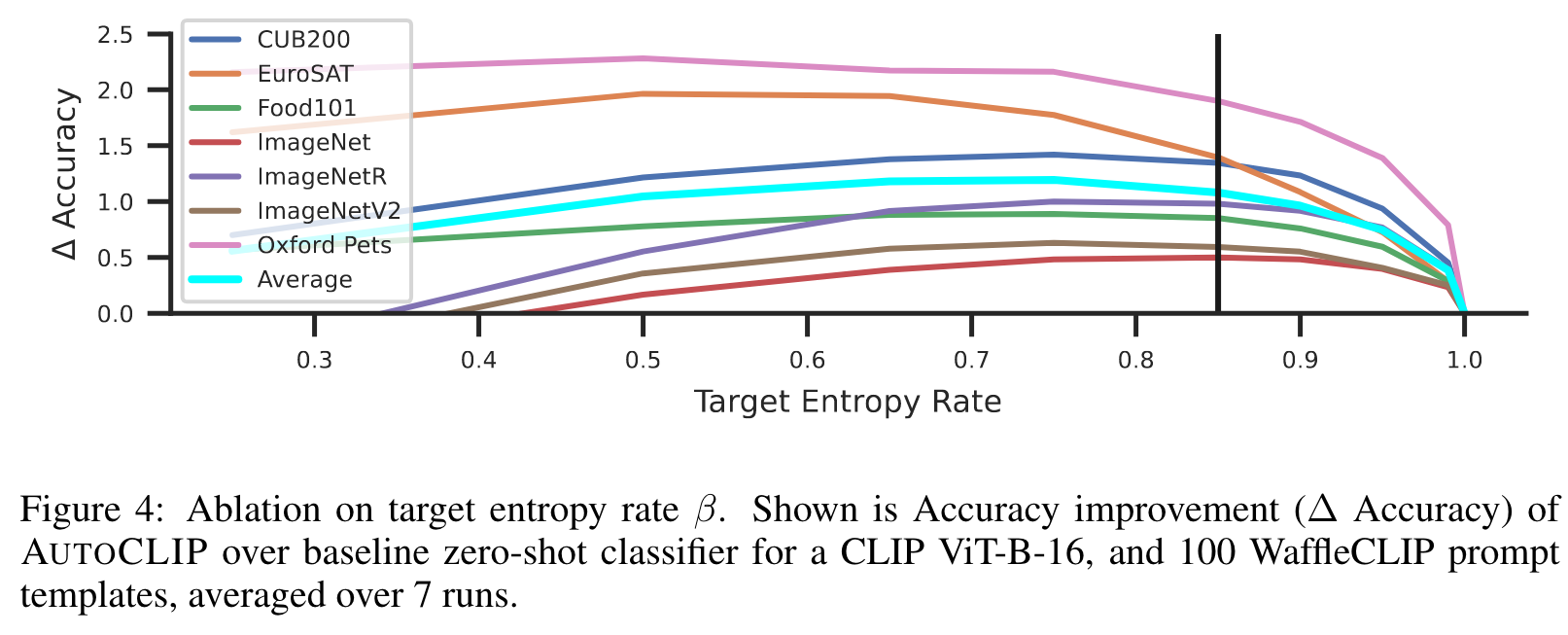In Figure 4, we observe that AUTOCLIP’s performance for most datasets does not depend strongly on the specific choice of the target entropy rate β as ∆ Accuracy stays relatively constant in the range β ∈ [0.7, 0.9]. (p. 8)

we empirically confirm that the logsumexp aggregation performs favorably compared to max/mean aggregation on all datasets. Moreover, it also outperforms entropy aggregation, which is a popular choice for test-time adaption (Wang et al., 2020; Shu et al., 2022). (p. 8)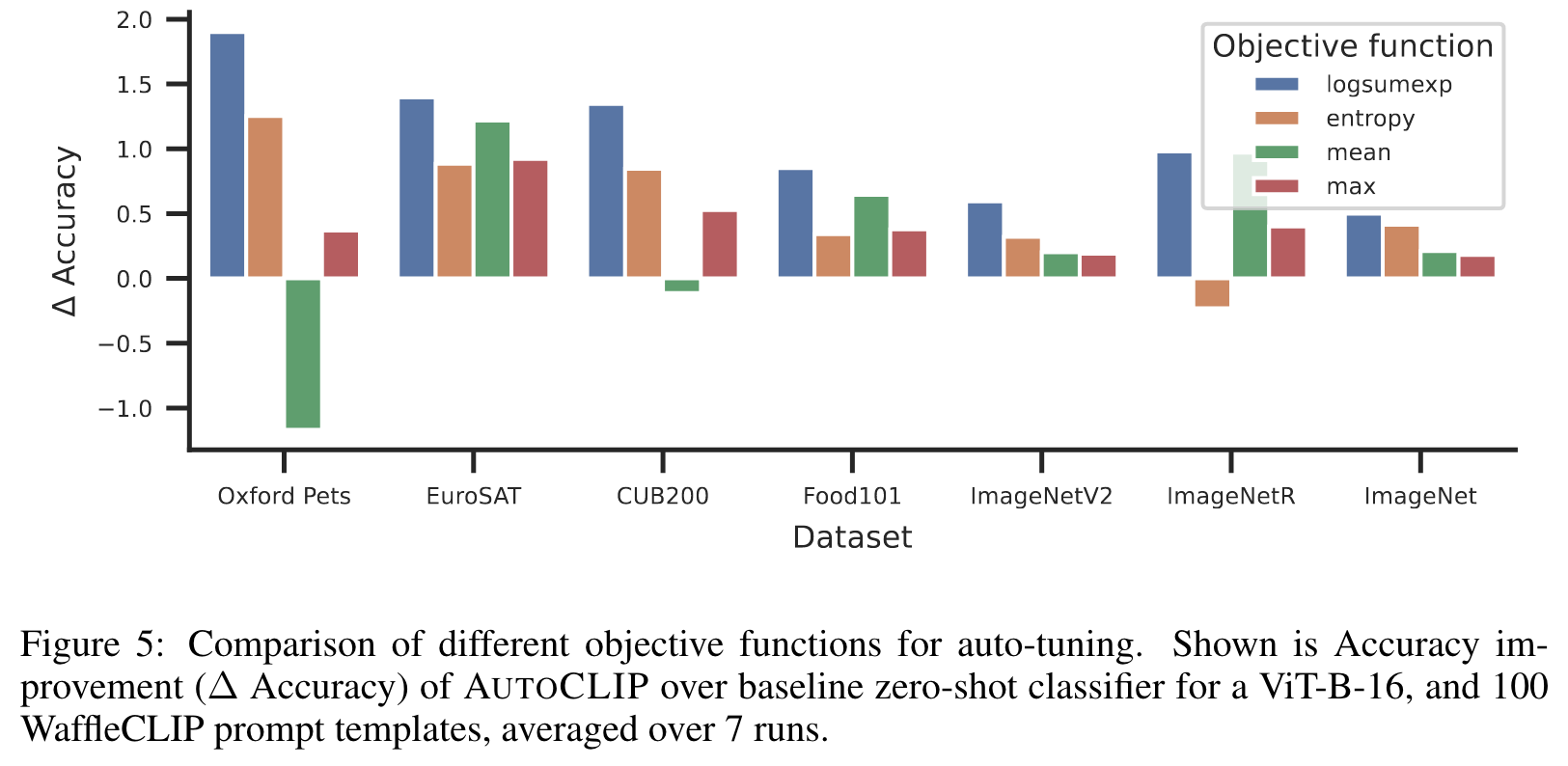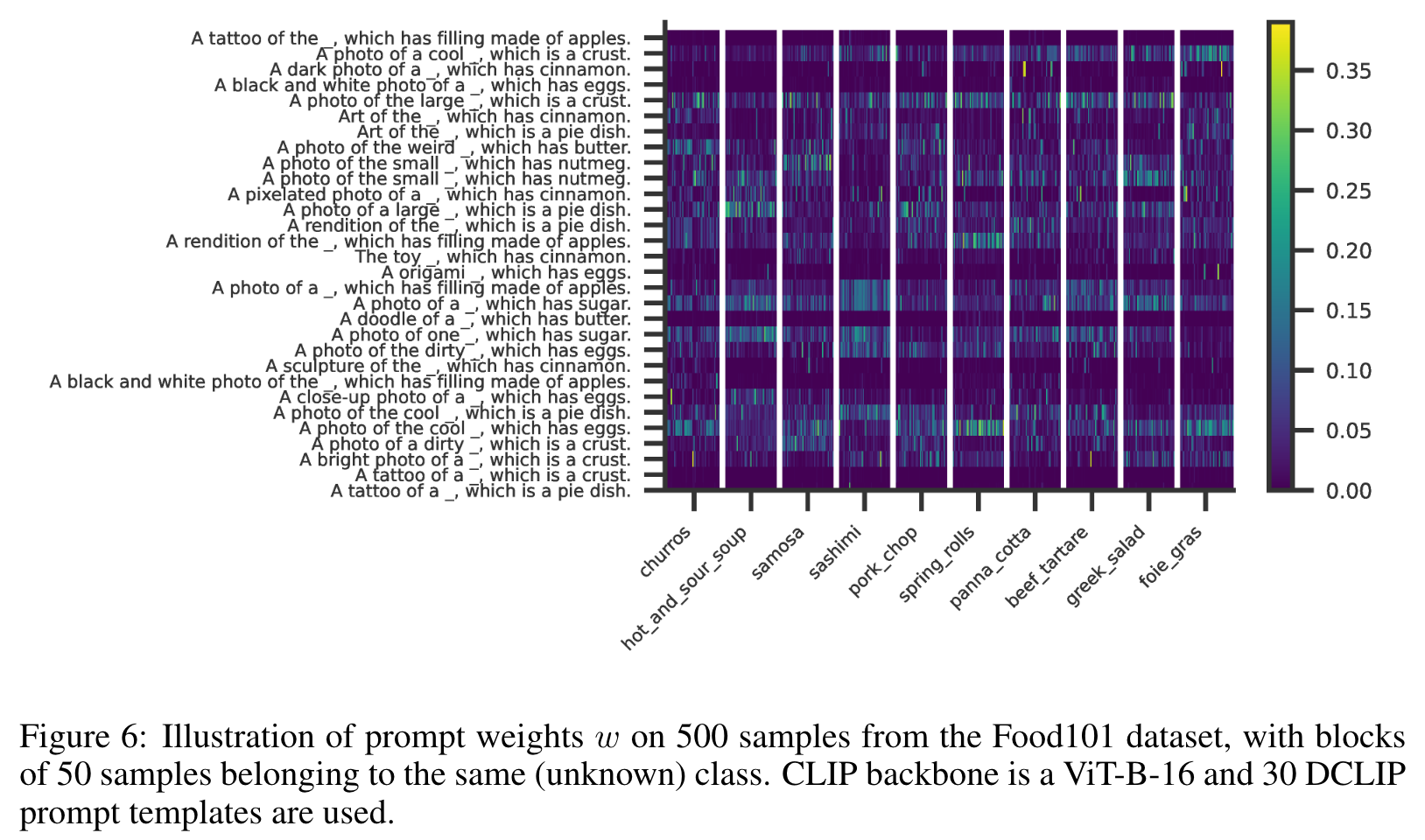Written on July 29, 2023